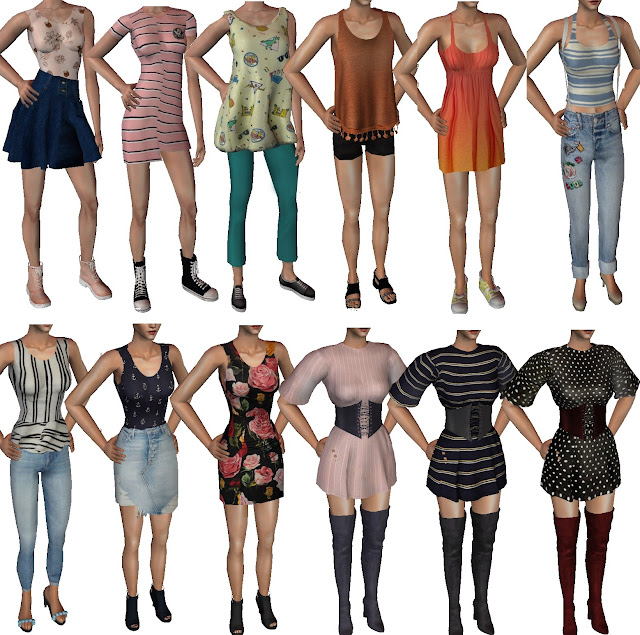The catalogue - FA Whole - Grecadea sims

1 HERE  2 HERE  3-5 HERE 6,7 HERE 8,9 HERE 10-12 HERE

1 and 2 HERE  3,4 and 5 HERE 6 HERE 7,8 HERE 9 HERE 10 HERE

1,2 HERE 3 HERE 4,5 HERE 6,7,8 HERE 9 HERE10 HERE

1 HERE, 2,3,4 HERE 5 HERE 6,7 HERE 8,9,10 HERE

1,2 HERE  3,4 HERE 5 HERE 6 HERE 7 HERE 8 HERE 9,10 HERE

1-3 HERE  4,5 HERE 6 HERE 7 HERE 8 HERE 9,10 HERE 11 HERE 12 HERE

1 HERE 2,3 HERE 4 HERE 5 HERE 6 HERE 7 HERE 8 HERE 9 HERE 10 HERE 11 HERE 12 HERE

1 HERE  2 HERE 3 HERE 4 HERE 5 HERE 6-8 HERE 9 HERE 10 HERE 11 HERE 12 HERE

1 HERE 2 HERE 3 HERE 4 HERE 5 HERE 6 HERE 7 HERE 8 HERE 9 HERE 10 HERE 11 HERE 12 HERE

1 HERE 2 HERE 3 HERE 4 HERE 5,6 HERE 7 HERE 8 HERE 9 HERE 10 HERE 11 HERE 12 HERE1 HERE 2 HERE 3 HERE 4,5 HERE 6-8 HERE 9 HERE 10,11 HERE 12 HERE1-3 HERE 4 HERE 5,6 HERE 7-9 HERE 10-12 HERE

1-6 HERE, 7 HERE, 8,9 HERE, 10,11 HERE, 12 HERE, 13,14 HERE

1-4 HERE, 5-8 HERE, 9-12 HERE 13-15 HERE

1-2 HERE 3-5 HERE, 6 HERE 7-10 HERE, 11 HERE, 12 HERE

1 HERE 2-3 HERE 4-7 HERE 8 HERE

#### You Might Also Like

1.2.